# Selina Concise Mathematics Class 6 ICSE Solutions Chapter 5 Natural Numbers and Whole Numbers

## Selina Concise Mathematics Class 6 ICSE Solutions Chapter 5 Natural Numbers and Whole Numbers (Including Patterns)

Selina Publishers Concise Mathematics Class 6 ICSE Solutions Chapter 5 Natural Numbers and Whole Numbers (Including Patterns)

### Natural Numbers and Whole Numbers Exercise 5A – Selina Concise Mathematics Class 6 ICSE Solutions

Question 1.
Fill in the blanks :
(i) Smallest natural number is …………..
(ii) Smallest whole number is …………
(iii) Largest natural number is …………
(iv) Largest whole number is ………..
(v) All natural numbers are …………
(vi) All whole numbers are not …………
(vii) Successor of 4099 is …………..
(viii) Predecessor of 4330 is …………….
Solution:Question 2.
Represent the following whole numbers on a number line :
0, 3, 5, 8, 10
Solution:Question 3.
State, true or false :
(i) Whole number are closed for addition.
(ii) If a and b are any two whole numbers, then a + b is not a whole number.
(iii) If a and b are any two whole numbers, then a + b = b + a
(iv) 0 + 18= 18 + 0
(v) Addition of whole numbers is associative.
(vi) 10 + 12 + 16 = (10 + 12) + 16 = 10 + (12 + 16)
Solution: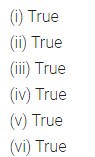Question 4.
Fill in the blanks :
(i) 54 + 234 = 234 + …………
(ii) 332 + 497 = ………… + 332
(iii) 286 + 0 = ………..
(iv) 286 x 1 = ……….
(v) a + (b + c) = (a + ………) + c
Solution: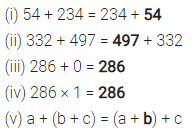Question 5.
By re-arranging the given numbers, evaluate :
(i) 237 + 308 + 163
(ii) 162 + 253 +338 + 47
(iii) 21 + 22 + 23 + 24 + 25 + 75 + 76 + 77 + 78 + 79
(iv) 1 + 2 + 3 + 4 + 596 + 597 + 598 + 599
Solution: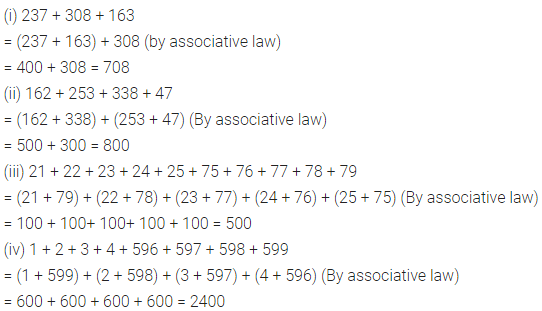Question 6.
Is a + b + c = a + (b + c) = (b + a) + c ?
Solution: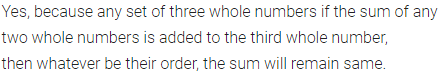Question 7.
Which property of addition is satisfied by :
(i) 8 + 7 = 15
(ii) 3+ (5 + 4) = (3 + 5)+ 4
(iii) 8 x (8 + 0) = 8 x 8 + 8 x 0
(iv) (7 + 6) x 10 = 7 x 10 + 6 x 10
(v) (15 – 12) x 18 = 15 x 18 – 12 x 18
(vi) 16 + 0= 16
(vii) 23 + (-23) = 0
Solution:Question 8.
State, True or False :
(i) The sum of two odd numbers is an odd number.
(ii) The sum of two odd numbers is an even number.
(iii) The sum of two even numbers is an even number.
(iv) The sum of two even numbers is an odd number.
(v) The sum of an even number and an odd number is odd number.
(vi) Every whole number is a natural number.
(vii) Every natural number is a whole number.
(viii) Every whole number + 0 = The whole number itself.
(ix) Every whole number x 1 = The whole number itself.
(x) Commutativity and associativity are properties of natural numbers and whole numbers both.
(xi) Commutativity and associativity are properties of addition for natural numbers and whole numbers both.
(xii) If x is a whole number then -x is also a whole number.
Solution: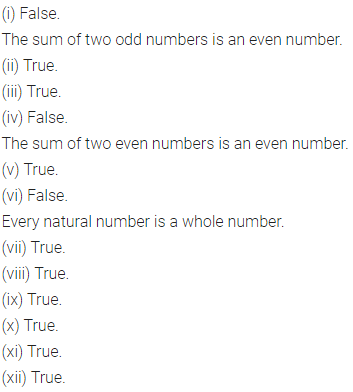### Natural Numbers and Whole Numbers Exercise 5B – Selina Concise Mathematics Class 6 ICSE Solutions

Question 1.
Consider two whole numbers a and b such that a is greater than b.
(i) Is a – b a whole number? Is this result always true?
(ii) b – a a whole number? Is this result always true?
Solution: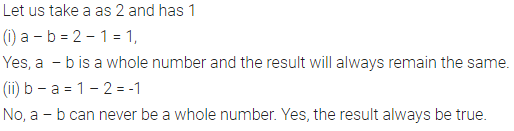Question 2.
Fill in the blanks :
(i) 8 – 0 = ……….. and 0 – 8 = ……….
8 – 0 ≠ 0 – 8, this shows subtraction of whole numbers is not ………..
(ii) 5 – 10 = ………., which is not a …………
⇒ Subtraction of ……….. is not closed.
(iii) 7 – 18 = ……….. and (7 – 18) – 5 = …………..
18 – 5 = …………. and (7 – 18) – 5 = ………….
Is (7 – 18) – 5 = 7 – (18 – 5) ?
⇒ Subtraction of whose numbers is not ………….
Solution:Question 3.
Write the identify number, if possible for subtraction.
Solution:Question 4.
Write the inverse, if possible for subtraction of whole numbers ?
Solution: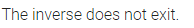Question 5.
12 × (9 – 6) = ………….. = ………….
12 × 9 – 12 × 6 = …………. = …………….
Is 12 × (9 – 6) = 12 × 9 – 12 × 6 ? ………….
Is this type of result always true ? ……………
Name the property used here …………..
Solution: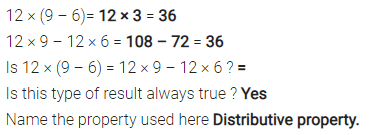Question 6.
(16 – 8) × 24 = ………….. = …………..
16 × 24 – 8 × 24 = …….. – ……….. = ………..
Is (16 – 8) × 24 = 16 × 24 – 8 × 24 ? …………..
Is the type of result always true? ………….
Name the property used here ………………
Solution: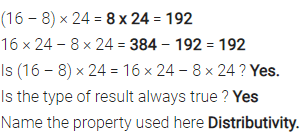### Natural Numbers and Whole Numbers Exercise 5C – Selina Concise Mathematics Class 6 ICSE Solutions

Question 1.
Fill in the blanks :
(i) 42 × o = ……….
(ii) 592 × 1 = ………..
(iii) 328 × 573 = ……….. × 328
(iv) 229 × ………… = 578 × 229
(v) 32 × 15 = 32 × 6 + 32 × 7 + 32 × ……….
(vi) 23 × 56 = 20 × 56 + ……… × 56
(vii) 83 × 54 + 83 × 16 = 83 × ( ……….. ) = 83 × ………….. = …………
(viii) 98 × 273 – 75 × 273 = ( …………. ) × 273 = ………… × 273
Solution:Question 2.
By re-arranging the given numbers, evaluate :
(i) 2 × 487 × 50
(ii) 25 × 444 × 4
(iii) 225 × 20 × 50 × 4
Solution:Question 3.
Use distributive law to evaluate :
(i) 984 × 102
(ii) 385 × 1004
(iii) 446 × 10002
Solution: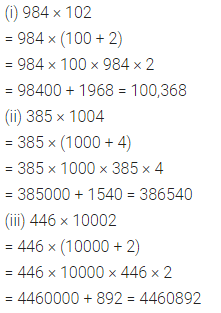Question 4.
Evaluate using properties :
(i) 548 × 98
(ii) 924 × 988
(iii) 3023 × 723
Solution:Question 5.
Evaluate using properties :
(i) 679 × 8 + 679 × 2
(ii) 284 × 12 – 284 × 2
(iii) 55873 × 94 + 55873 × 6
(iv) 7984 × 15 – 7984 × 5
(v) 8324 × 1945 – 8324 × 945
(vi) 3333 × 987 + 13 × 3333
Solution: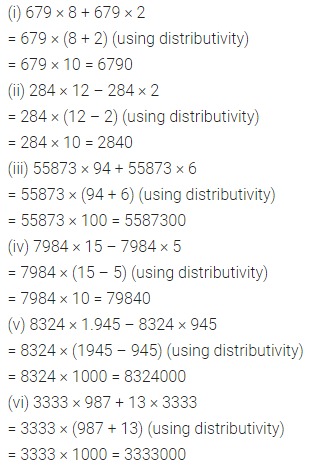Question 6.
Find the product of the :
(i) greatest number of three digits and smallest number of five digits.
(ii) greatest number of four digits and the greatest number of three digits.
Solution: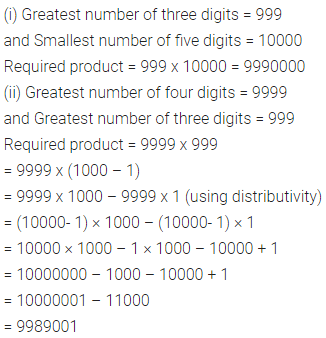Question 7.
Fill in the blanks :
(i) (437 + 3) × (400 – 3) = 397 × ……….
(ii) 66 + 44 + 22 = 11 × (………..) = 11 × ……….
Solution:Question 8.
Evaluate :
(i) 355 × 18
(ii) 6214 × 12
(iii) 15 × 49372
(iv) 9999 × 8
Solution:### Natural Numbers and Whole Numbers Exercise 5D – Selina Concise Mathematics Class 6 ICSE Solutions

Question 1.
Show that :
(i) division of whole numbers is not closed
(ii) any whole number divided by 1, always gives the number itself.
(iii) every non-zero whole number divided by itself gives 1 (one).
(iv) zero divided by any non-zero number is zero only.
(v) a whole number divided by 0 is not defined.
For each part, given above, give an example.
Solution: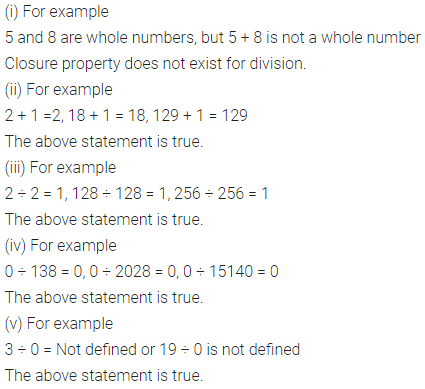Question 2.
If x is a whole number such that x ÷ x = x, state the value of x.
Solution: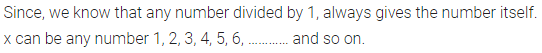Question 3.
Fill in the blanks :
(i) 987 + 1 = …………
(ii) 0 + 987 = ……….
(iii) 336 – (888 + 888) = ……….
(iv) (23 + 23) – (437 + 437) = ………
Solution: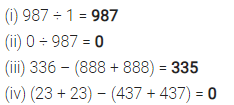Question 4.
Which of the following statements are true ?
(i) 12 ÷ (6 × 2) = (12 ÷ 6) × (12 ÷ 2)
(ii) a ÷ (b – c) = $$\frac { a }{ b } -\frac { a }{ c }$$
(iii) (a – b) ÷ c = $$\frac { a }{ c } -\frac { b }{ c }$$
(vi) (15 – 13) ÷ 8 = (15 ÷ 8) – (13 ÷ 8)
(v) 8 ÷ (15 – 13) = $$\frac { 8 }{ 15 } -\frac { 8 }{ 13 }$$
Solution:### Natural Numbers and Whole Numbers Exercise 5E – Selina Concise Mathematics Class 6 ICSE Solutions

Question 1.
Find the difference between the largest number of four digits and the smallest number of six digits.
Solution: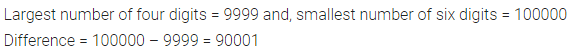Question 2.
Find the difference between the smallest number of eight digits and the largest number of five digits.
Solution:Question 3.
The product of two numbers is 528. If the product of their unit’s digits is 8 and the product of their ten’s digits is 4 ; find the numbers.
Solution: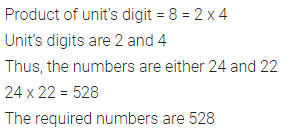Question 4.
Does there exist a number a such that a ÷ a = a?
Solution:Question 5.
Divide 5936 by 43 to find the quotient and remainder. Also, check your division by using the formula,
dividend = divisor × quotient + remainder.
Solution:### Natural Numbers and Whole Numbers Exercise 5F – Selina Concise Mathematics Class 6 ICSE Solutions

Question 1.
(i) 1 × 9 + 1 = 10
12 × 9 + 2 = 110
123 × 9 + 3 = 1110
(ii) 9 × 9 + 7 = 88
98 × 9 + 6 = 888
987 × 9 + 5 = 8888
(iii) 1 × 8 + 1 = 9
12 × 8 + 2 = 98
123 × 8 + 3 = 987
(iv) 111 ÷ 3 = 37
222 ÷ 6 = 37
333 ÷ 9 = 37
Solution: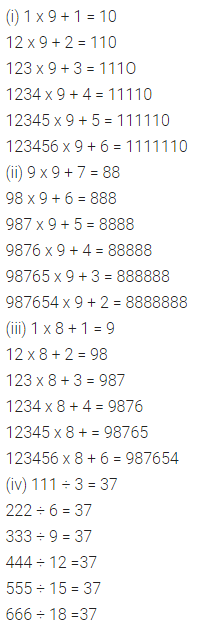Question 2.
Complete each of the following magic squares :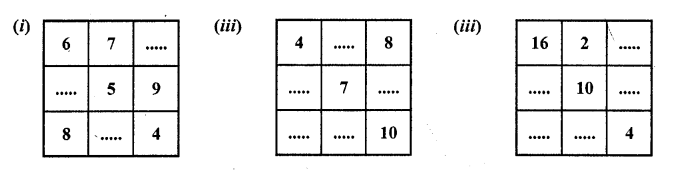Solution: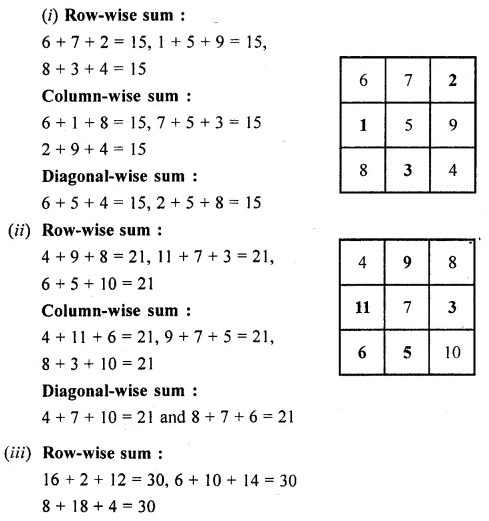Question 3.
See the following pattern carefully :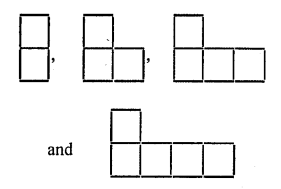(i) If n denotes the number of figure and S denotes the number of matches ; find S in terms of n.
(ii) Find how many matches are required to make the :
(1) 15th figure
(2) 40th figure
(iii) Write a discretion of the pattern in words.
Solution: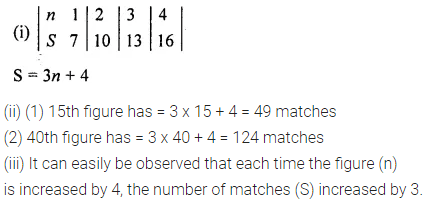Question 4.
(i) In the following pattern, draw the next two figures.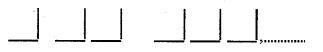(ii) Construct a table to describe the figures in the above pattern.
(iii) If n denotes the number of figure and L denotes the number of matches, find L in terms of n.
(iv) Find how may matches are required to make the :
(1) 12th figure
(2) 20th figure
Solution:Question 5.
In each of the following patterns, construct next figure.
(i) In each case, if n denotes the number of figure and F denotes the number of matchsticks used, find F in terms of n.
(ii) Also find, in each case, how many matches are required to make the : 16th figure and 30th figure.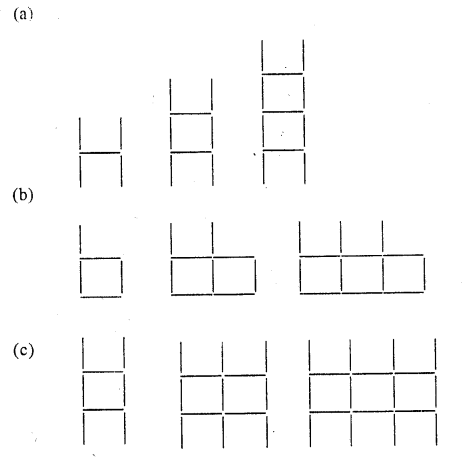Solution: